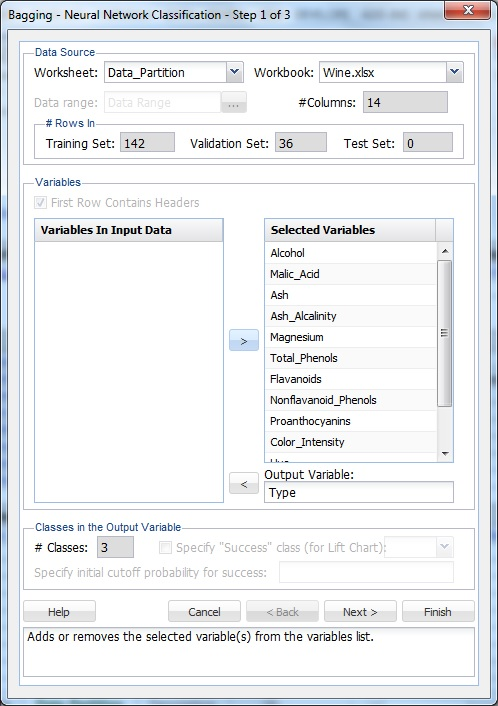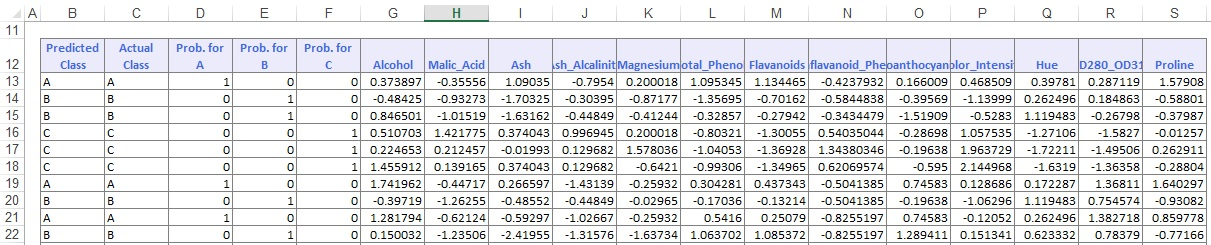XLMiner provides four options when creating a Neural Network classifier: Boosting, Bagging (ensemble methods), Automatic, and Manual. This example focuses on creating a Neural Network using the bagging ensemble method. This example illustrates how to create a Neural Network using a manual architecture and an automatic architecture.

On the XLMiner ribbon, from the Data Mining tab, select a cell on the Data_Partition worksheet, then select Classify - Neural Network - Bagging to open the Bagging - Neural Network Classification - Step 1 of 3 dialog.At Output Variable, select Type, and from the Selected Variables list, select all remaining variables. Since the Output Variable contains three classes (A, B, and C) to denote the three different wineries, the options for Classes in the Output Variable are disabled. The options under Classes in the Output Variable are only enabled when the number of classes is equal to 2.

Click Next to advance to the Step 2 of 3 dialog.The option Normalize input data is selected by default. Normalizing the data (subtracting the mean and dividing by the standard deviation) is important to ensure that the distance measure accords equal weight to each variable. Without normalization, the variable with the largest scale would dominate the measure.

Leave the Number of weak learners at the default of 50. This option controls the number of weak classification models that are created. The ensemble method stops when the number or classification models created reaches the value set for the Number of weak learners. The algorithm then computes the weighted sum of votes for each class, and assigns the winning classification to each record.

For Neuron weight initialization seed, leave the default integer value of 12345. XLMiner uses this value to set the neuron weight random number seed. Setting the random number seed to a non-zero value ensures that the same sequence of random numbers is used each time the neuron weight are calculated. If left blank, the random number generator is initialized from the system clock, so the sequence of random numbers is different in each calculation. If you need the results from successive runs of the algorithm to another to be strictly comparable, enter a value for Set seed.

At Bootstrapping random seed, leave the default integer value of 12345. Setting the random number seed to a non-zero value ensures that the same sequence of random numbers is used each time the Training Set is selected. If left blank, the random number generator is initialized from the system clock, so the sequence of random numbers is different in each calculation. To return comparable results from successive runs of the algorithm, enter a value at Set seed.

At # Hidden Layers, keep the default setting of 1, and at # Nodes Per Layer, keep the default setting of 12. Since # hidden layers (max 4) is set to 1, only the first text box is enabled.

At # Epochs, keep the default setting of 30. An epoch is one sweep through all records in the Training Set.

For Gradient Descent Step Size, keep the default setting of 0.1. This is the multiplying factor for the error correction during backpropagation; it is roughly equivalent to the learning rate for the neural network. A low value produces slow learning, a high value produces rapid (but erratic) learning. Values for the step size typically range from 0.1 to 0.9.

For Weight Change Momentum, keep the default setting of 0.6. In each new round of error correction, some memory of the prior correction is retained so that an outlier that crops up does not spoil accumulated learning.

For Error Tolerance, keep the default setting of 0.01. The error in a particular iteration is backpropagated only if it is greater than the error tolerance. Typically, error tolerance is a small value in the range from 0 to 1.

For Weight Decay, keep the default setting of 0. To prevent over-fitting of the network on the Training Set, enter a weight decay to penalize the weight in each iteration. Each calculated weight is multiplied by (1-decay).

Nodes in the hidden layer receive input from the input layer. The output of the hidden nodes is a weighted sum of the input values. This weighted sum is computed with weights that are initially set at random values. As the network learns these weights are adjusted. This weighted sum is used to compute the hidden node's output using a transfer function, or activation function. Select Standard (default setting) to use a logistic function for the activation function with a range of 0 and 1. This function has a squashing effect on very small or very large values, but is almost linear in the range where the value of the function is between 0.1 and 0.9. Select Symmetric to use the tanh (hyperbolic tangent) function as the transfer function, the range being -1 to 1. For this example, keep the default selection of Standard. If more than one hidden layer exists, this function is used for all layers.

As in the hidden layer output calculation, the output layer is also computed using the same transfer function. Select Standard (default setting) to use a logistic function for the transfer function with a range of 0 and 1. Select Symmetric to use the tanh function for the transfer function, the range being -1 to 1. Select Softmax to use a generalization of the logistic function that maps a length-p vector of real values to a length-K vector of values. In neural networks, the Softmax function is often implemented at the final layer of a classification neural network to impose the constraints that the posterior probabilities for the output variable must be >= 0 and <= 1, and sum to 1. For this example, keep the default of Standard.

XLMiner V2015 provides the ability to partition a data set from within a classification or prediction method by selecting Partitioning Options on the Step 2 of 3 dialog. If this option is selected, XLMiner partitions data set immediately before running the prediction method. If partitioning has already occurred on the data set, this option is disabled. For more information on partitioning, please see the Data Mining Partition section.

Click Next to advance to the Bagging - Neural Network Classification - Step 3 of 3 dialog.Under Score Training Data and Score Validation Data, Summary Report is selected by default. Under both Score Training Data and Score Validation Data, select Detailed Report. Lift Charts are disabled when the number of classes is greater than 2. Since a Test Data partition was not created, the options under Score Test Data are disabled.

For more information on the Score New Data options, see the Scoring New Data section.

Click Finish to view the output. Click the NNCBag_Output worksheet to view the Output Navigator.Scroll down to the Classification Matrices to view the Neural Network Classification algorithm performed. The algorithm finished with two errors in the Training Set and one error in the Validation Set, resulting in an overall error of 1.41% in the Training Set, and 2.78% in the Validation Set. These small errors indicate that the ensemble method model is a good fit to the data.Click the NNCBag_TrainScore to open the Classification of the Training Data and view the Predicted class assignment versus the Actual class to which each record in the Training Set is assigned, along with the probability for each class. The class with the largest probability becomes the assigned class.Click the NNCBag_ValidScore to open the Classification of the Validation Data and view the Predicted Class assignment versus the Actual class for each record in the Validation Set. The class with the largest assigned probability becomes the assigned class.For information on scoring new data, see the Stored Model Sheet, NNC_Stored1.

With this particular data set, the models created by both ensemble methods appear to be a good fit to the data.